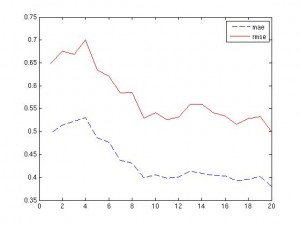The last few days saw me experimenting with one particular data set and different parameters of the SVM regression model for those data. For the data set at hand, I figured epsilon, the width of the error pipe to be 0.3 and the standard deviation of the rbf kernel to be 12. Other kernels won’t work on those data and I’ll have to do a comparison of those results with the number of support vectors that are a further parameter that constitutes the models.

The first figure shows one of the trial runs that aims at determing epsilon. It was varied from 1.0 (0) to 0.05 (20) in 20 steps (hence the x-scale that I haven’t labeled). In those runs the minimum was around 15, which means epsilon = 0.3.The second figure shows one of the trial runs that aims at determining the standard deviation of the rbf kernel. Here, it was simply varied from 1 to 20 and the results are shown. The minimum is somewhere around 12.Finally, the matlab script that produced the second figure, fully commented. It’s already linked in the static page on the left.